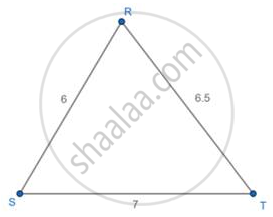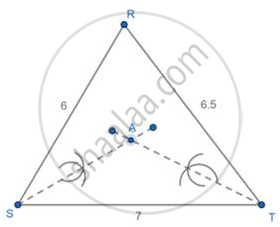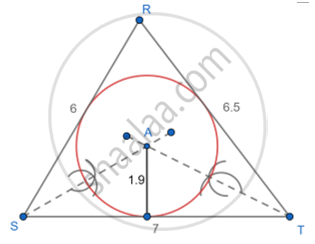# Construct the Incircle of δ Rst in Which Rs = 6cm, St 7 Cm, Rt = 6.5 Cm. - Geometry

Sum

Construct the incircle of Δ RST in which RS = 6cm, ST 7 cm, RT
= 6.5 cm.

#### Solution

1: Draw a Δ RST with the given measurements.2: Construct the angle bisector of any two angles (S and T) and let them meet at A(center of inscribed circle).3: With A as an external point drop a perpendicular to any one of the sides to meet at D.4: With A as center and AD as radius draw the circle this circle touches all the sides of the triangle.Concept: Inscribed Angle
Is there an error in this question or solution?